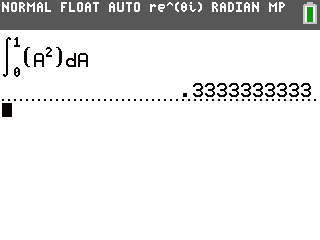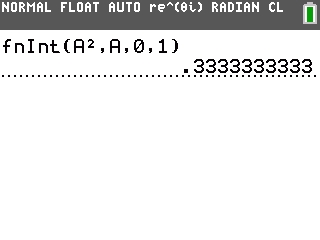# Knowledge Base

## Solution 34694: Using the fInt Function Integral Command on the TI-84 Plus Family of Graphing Calculators.

### How do I use the fInt Function Integral command on the TI-84 Plus family of graphing calculators?

The fnInt command returns the numerical integral of an expression with regards for real numbers only. The numerical integral is based on the Gauss-Kronrod method. The numerical integral is calculated with respect to the variable, given a lower limit, upper limit and a tolerance. Tolerance is optional and if not specified it is defaulted to 1E-5. The TI-84 Plus family of graphing calculators display a template when in MATHPRINT MODE but while in this model Tolerance cannot be entered.

When in CLASSIC mode, the syntax for the fnInt command is below:

fnInt(expression, variable, lower, upper[,tolerance]), between lower and upper, with specified tolerance.

Example: Find the function integral of A2, for the variable A, with 0 as the lower bound and 1 as the upper bound using the MATHPRINT mode:

1) Press [MATH] then  to select 9:fInt(
2) Press  [→]  [→] [alpha] [math] [x²] [→] [alpha] [math] [enter]In CLASSIC mode, follow the steps below:

1) From the main screen press [math] then  to select 9:fInt(
2) Press [alpha] [math] [x2] [,] [alpha] [math] [,]  [,]  [)] [enter]